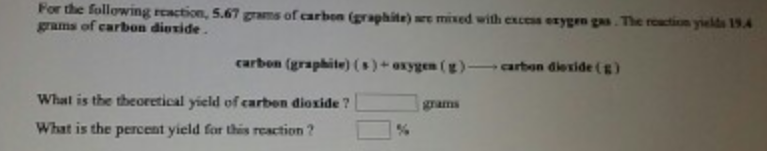# Problem: For the following reaction, 5.67 grams of carbon (graphite) are mixed with excess oxygen gas. The reaction yields 19.4 grams of carbon dioxide. carbon (graphite) (s) + oxygen (g) → carbon dioxide (g) What is the theoretical yield of carbon dioxide? What is the percent yield for this reaction?

###### FREE Expert Solution
100% (372 ratings)###### Problem Details

For the following reaction, 5.67 grams of carbon (graphite) are mixed with excess oxygen gas. The reaction yields 19.4 grams of carbon dioxide.

carbon (graphite) (s) + oxygen (g) → carbon dioxide (g)

What is the theoretical yield of carbon dioxide?

What is the percent yield for this reaction?